Related Articles
Perspective Projection and its Types
• Last Updated : 07 Aug, 2020

In Perspective Projection the center of projection is at finite distance from projection plane. This projection produces realistic views but does not preserve relative proportions of an object dimensions. Projections of distant object are smaller than projections of objects of same size that are closer to projection plane. The perspective projection can be easily described by following figure :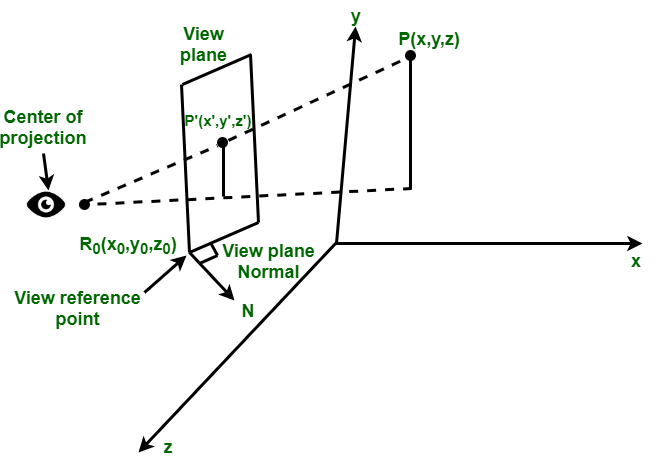1. Center of Projection –
It is a point where lines or projection that are not parallel to projection plane appear to meet.
2. View Plane or Projection Plane –
The view plane is determined by :

• View reference point R0(x0, y0, z0)
• View plane normal.

3. Location of an Object –
It is specified by a point P that is located in world coordinates at (x, y, z) location. The objective of perspective projection is to determine the image point P’ whose coordinates are (x’, y’, z’)

Types of Perspective Projection :
Classification of perspective projection is on basis of vanishing points (It is a point in image where a parallel line through center of projection intersects view plane.). We can say that a vanishing point is a point where projection line intersects view plane.

The classification is as follows :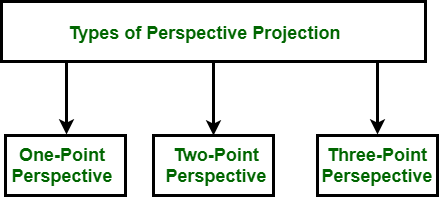• One Point Perspective Projection –
One point perspective projection occurs when any of principal axes intersects with projection plane or we can say when projection plane is perpendicular to principal axis.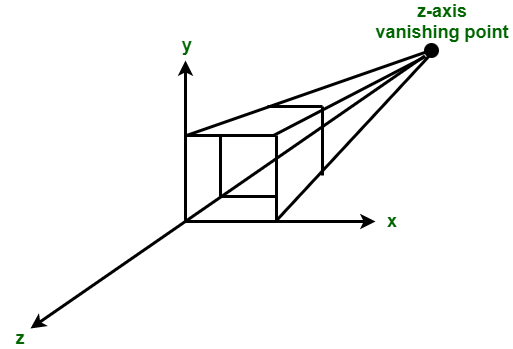In the above figure, z axis intersects projection plane whereas x and y axis remain parallel to projection plane.

• Two Point Perspective Projection –
Two point perspective projection occurs when projection plane intersects two of principal axis.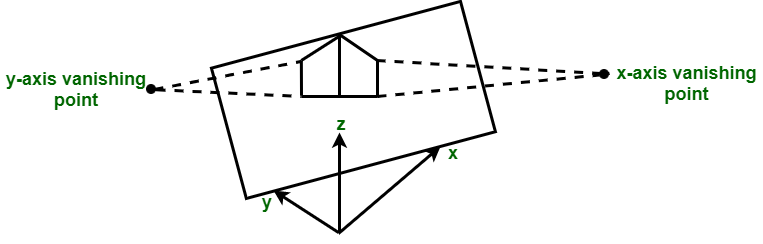In the above figure, projection plane intersects x and y axis wheres z axis remains parallel to projection plane.

• Three Point Perspective Projection –
Three point perspective projection occurs when all three axis intersects with projection plane. There is no any principle axis which is parallel to projection plane.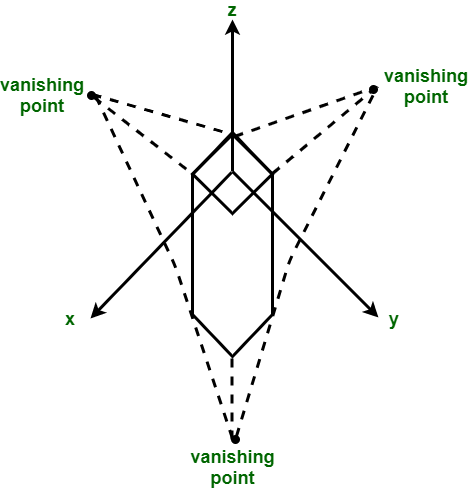Application of Perspective Projection :
The perspective projection technique is used by artists in preparing drawings of three-dimensional objects and scenes.

Attention reader! Don’t stop learning now. Get hold of all the important DSA concepts with the DSA Self Paced Course at a student-friendly price and become industry ready.

My Personal Notes arrow_drop_up